International Journal of Systems Science and Applied Mathematics
Volume 1, Issue 1, May 2016, Pages: 1-7

Case Report

Estimates of Evaporation from Reservoirs Using ANN Model, a Case Study of Amir Kabir Dam, Karaj City, Iran

1Nature Engineering, Faculty of New Sciences and Technologies, University of Tehran, Tehran, Iran

2Faculty of Environment, University of Tehran, Tehran, Iran

3Faculty of New Sciences and Technologies, University of Tehran, Tehran, Iran

4Environmental Planning, Faculty of Environment, University of Tehran, Tehran, Iran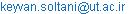(K. Soltani)

*Correspondence author

Keyvan Soltani, Ahmad Nohegar, Mohammad Hossein Jahangir, Seyed Javad Sadatinejad, Shahrzad Bouzari. Estimates of Evaporation from Reservoirs Using ANN Model, a Case Study of Amir Kabir Dam, Karaj City, Iran. International Journal of Systems Science and Applied Mathematics. Vol. 1, No. 1, 2016, pp. 1-7. doi: 10.11648/j.ijssam.20160101.11

Received: April 21, 2016; Accepted: April 28, 2016; Published: May 6, 2016

Abstract: Evaporation as a natural parameter due to the release of water from the upper part of mankind have always been of interest to scholars and researchers. In this study, we try to apply the artificial neural network model to estimate evaporation from Amir Kabir dam, the accuracy of the model is evaluated. In this context, the number 17 in the 1997 to 2014 solar years were used and consecutive errors after the procedure and the amount of evaporation from the surface of the dam structure was selected Amir Kabir The structure of the first and the second layer 7 and 8 neurons with 100 replicates to calculate it, the best results were obtained. Coefficients obtained from statistical analysis using ANN networks were considered in selecting the best structure the correlation coefficient of 90% in 0.0112 error was calculated. To determine the parameters of the evaporation rate at 17 years of data available, importing and using MATLAB software cubic best fit through the points in the data was drawn. Mann-Kendall method as well as the routing of data and trend parameters were determined the test statistics for 15 years between 1997 to 2014 solar years, and then the resulting cubic method was compared.

Keywords: ANN, Evaporation, Correlation Coefficient, Mann-Kendall, Amir Kabir Dam

Contents

1. Introduction

The primary and essential element showing dryness level in a region is the relation between annual precipitation and environmental water evaporation potential. Reservoirs’ evaporation modeling is important in order to predict surface evaporation level, water loss of evaporation, inaccessible water, proper and practical planning in reducing the amount of this evaporation, as well as economic assessment. Through this prediction, economic and practical decisions made to reduce evaporation and consequently increase accessible water level, production and efficiency (Ma et al. 2011). However, conventional statistical and experimental models are fundamental instruments of representing hydrologic variables and understanding the rules governing on a system, they have many limitations making models’ function difficult and costly to the users (Rajaei et al. 2010). One of these limitations is the need for accurate and diverse information to estimate understudied parameter. Heavy and time-consuming computations, particularly in haphazard phenomena, caused many problems for experts and users (Nourani and Sayahfard, 2014). Therefore, the experts intended to save time and cost through applying intelligent models and acquire desired results at the right time from the existing data (Tabesh and Dini, 2010). Of these useful and smart tools is using artificial neural network (ANNs) that intelligently achieves desired output through establishing a proper relation between input variables in the shortest possible time and output tools and provides the results to the experts at best (Soltani, A. 1998). Some studies conducted about this issue including studying the effect of different compositions of climatic parameters on dam reservoir evaporation loss by Deswall and Paul (2008). In addition, an ANN model applied to estimate the amount of existing water evaporation in greenhouse soil in China, the results of which demonstrate its high preciseness. Tabarie et al also used ANN and regression models to estimate the amount of pan evaporation in a semiarid climate region in Iran. The results revealed the superiority of ANN network model in relatively real estimation comparing regression model (Tabari et al. 2010).

2. Study Area

Amir Kabir Dam, constructed in 1958-1963, geographically located in northeastern of Karaj city on Chalus road and Khuzankola area in the Variyan strait. This dam with 390 m crest length and 180 m height created about 4km lake. Amir Kabir Dam with 107 steel towers is a multi-purpose dam watered by Karaj River mainstream traveling a 75 km mountainous distance from north of Karaj city toward north-to-south direction.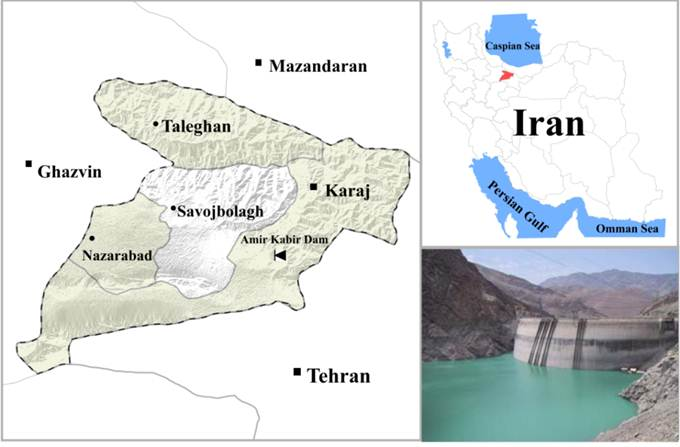Fig. 1. Location of Amir Kabir Dam in Iran.

3. Methodology

This study used average rainfall (mm), temperature in Celsius (oc), wind speed in meter/second, and lake area in km2; all information, in monthly scale, were main inputs of the artificial network. Following figures represent monthly data of artificial network within 1997-2014 through using Matlab software; the best fitted offered using Cubic method.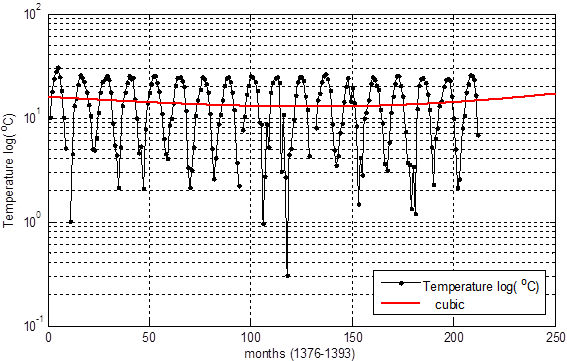Fig. 2. Changes in air temperature (°C) within years of 1997-2014.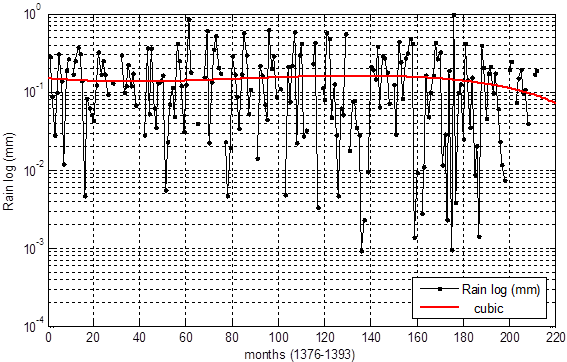Fig. 3. Changes in precipitation (mm) within years of 1997-2014.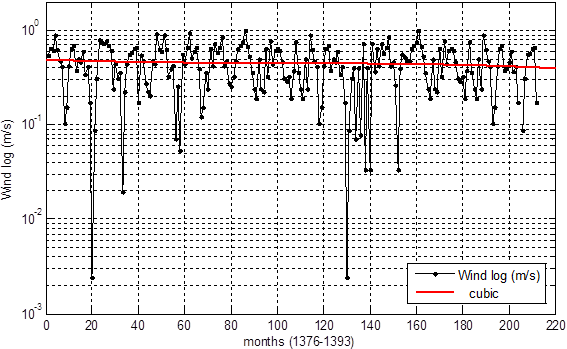Fig. 4. Changes in wind speed (m/s) within 1997-2014.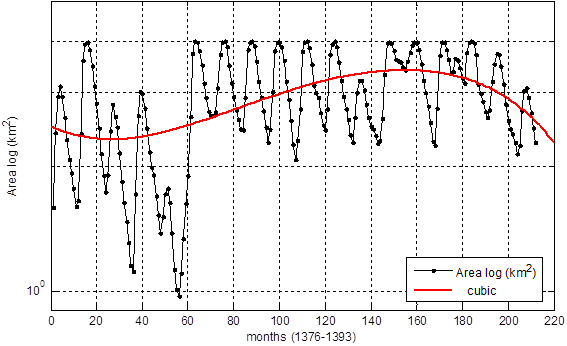Fig. 5. Changes in lake area (km2) within 1997-2014.

As figures show, changes in wind speed and temperature were constant during the years; while, precipitation changes were more tangible over years. Further, lake area changed and reduced more quickly. In addition, evaporation change trends also studied by Cubic method as follows.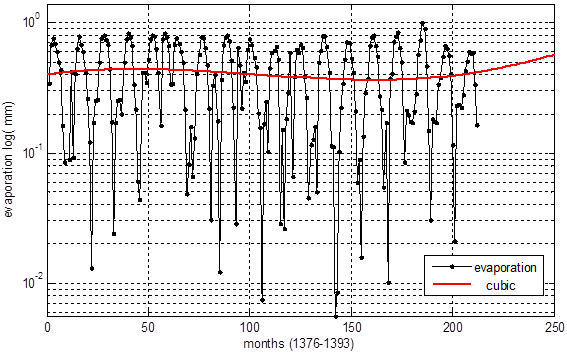Fig. 6. Changes in evaporation (mm) within 1997-2014.

The figure shows that changes in evaporation are relatively constant in spite of large variations over years. However, Mann-Kendall test applied to better determine changing trend of dependent parameters of evaporation.

3.1. Trend Analysis of Evaporation and Effective Parameters Using Mann-Kendall Method

Mann-Kendall instability statistic was used for significance test and mutation detection in time series. Mann-Kendall statistics is mainly applied in environmental sciences, as these are robust and simple tests controlling under threshold values (Jahanbakhshasl et al, 2012).

Mann-Kendall test steps are as follows:

Data are chronologically ordered in term of n.

Data ranked using T statistics (i rank to the prior rank).

Mathematical expectation (Ei), variance (Vi), and Mann-Kendall index (Ui) calculated using the following numerical relations: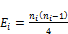(1)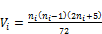(2)(3)

Where, ni is data time series.

Partial and short-term routes of mutation and initiation of time series from time series chart were used in term of U and Uvalues. Time series chart of U and Usuccessive statistics values computed by relations 3 and 6.

3.2. U Computation Steps

Ranked data that used Tstatistics (i rank to the succeeding rank).

Mathematical expectation Ei, variance Vi, and the index Ui’ obtained through the following relations.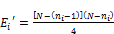(4)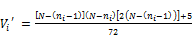(5)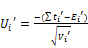(6)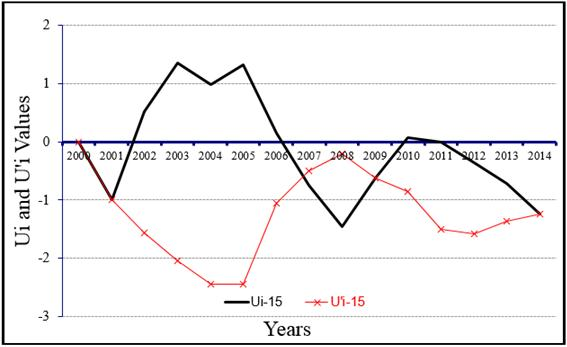Fig. 7. Annual changes in wind speed by Mann-Kendall method.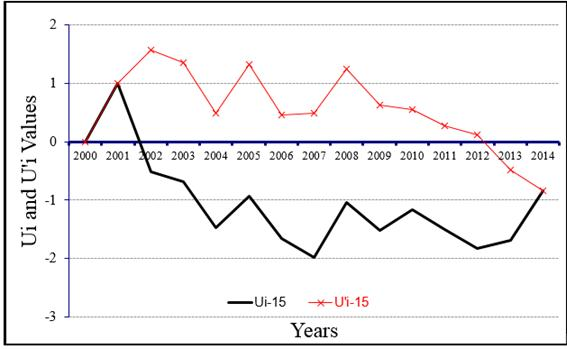Fig. 8. Annual change trend in temperature by Mann-Kendall method.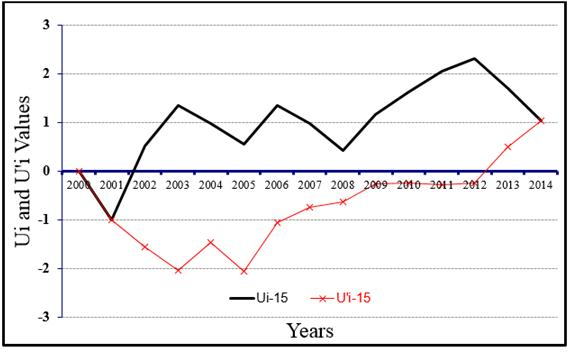Fig. 9. Annual changes in lake area by Mann-Kendall method.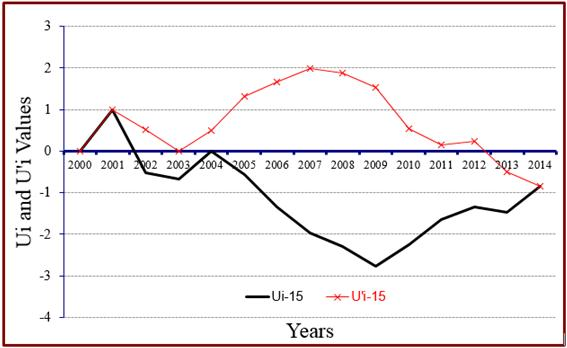Fig. 10. Annual change trend in evaporation by Mann-Kendall method.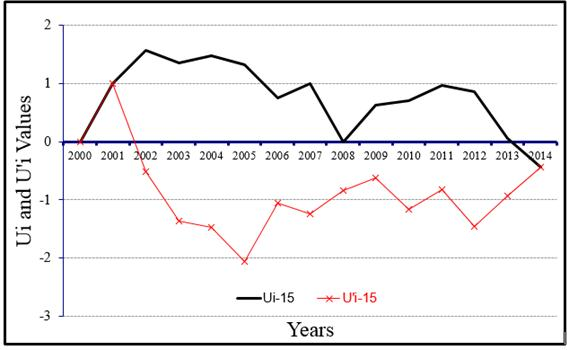Fig. 11. Annual change trend in rainfall by Mann-Kendall method.

Where, N is the numbers of statistic years. The intersection of U and Uindices at confidence level of 95% shows changes in time series and U behavior after intersection represents the series trend status (descending or ascending). Nonintersecting of the two factors indicates lack of series

The Mann-Kendall test for trend showed that figures of annual changes in temperature, wind speed, and rainfall lack the trend, in which -1.96<U<1.96 indicating lack the trend in data. U value of changes in lake area figure, within 2010- 2012, did not exceed 1.96 revealing the increasing trend in this period, which again follows a descending trend. This is also seen in Cubic method. U chart in annual trend of changes in evaporation through Mann-Kendall method within 2007-2011 exceeded the -1.96 range indicating the negative trend in this period.

3.3. Artificial Neural Network

An artificial neural network consisted of three main layers of input, hidden (middle layer), and output layer. As the following figure shows, the existing information are given to the system as the input layer; this layer, indeed, serves as a transferring layer and is a tool for data introduction to the system (Traore et al, 2010). The layer giving analysis results is the output layer, which is in fact the modeling stage. The middle layer acts as the model processor; processor nodes are at this stage (Ma et al. 2011). It needs considering that the numbers of hidden layers and nodes obtained using trial and error. Multilayer perceptron model (MLP) is one of the simplest and efficient recommended arrays for modeling real nerves. It is consisted of one input layer, one or several hidden layers, and an output layer. In this structure, all neurons of a layer connected to all neurons of the next layer; this array forms a so-called network with complete connections. The following figure illustrates schematic of a three-layer perceptron network (Reddy, 2006).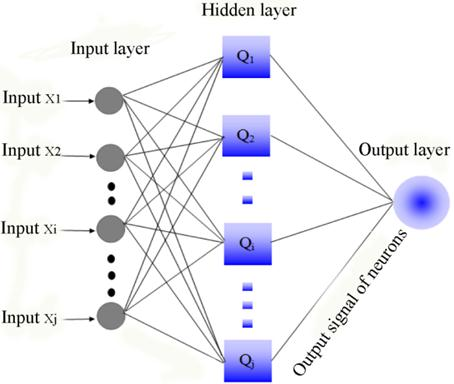Fig. 12. Structure of artificial neural network with three input, output, and middle (hidden) layers.

It easily inferred that neuron numbers in each layer is independent of neuron numbers in other layers. In this method, weight changing in any step is:(7)

Where, η is the weights’ modification constant determining algorithm convergence rate (Zoqi and Saeidi, 2011).

In order to find the amount of weights’ modification the following formula is used per stage, which is error derivation in weights.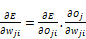(8)

It evidently seen that output of nerve stimulation function only depends on input and corresponding weights. Given that the output function is sigmoid, the output of jth nerve written as follows: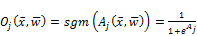(9)

Network total error in the form of sum of output layer neurons can be written as follows: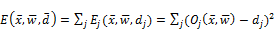(10)

Finally, considering that artificial neural network embraces three input, output, and hidden layers, the final relation of weights correction is as follows (Zoqi and Saeedi, 2011):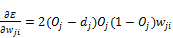(11)

Modeling in neural network performed through using aforementioned formulas and several other complex formulas, it also gives the best-fitted output to our inputs.

The following table represents neural network defaults including neuron numbers and nodes of hidden layers. Correlation coefficient and MSE error of any default are also shown in the table. The best proper number of neural network defaults with maximum correlation coefficient and minimum error also selected. It is necessary notifying that regarding changes in the numbers of layers, neurons, and replications up to one hundred times; therefore, only the structures mentioned with acceptable correlation coefficient and error excluding all stages.

Table 1. Replicas and measured values of MSE and R2 for any optimum structure.

 Raw Layer structure Replication Training Validation Test Total MSE R2 MSE R2 MSE R2 MSE R2 1 9-3-1 80 0.0229 81 0.0131 87 0.0149 90 0.013 83 2 6-10-1 100 0.032 88 0.0194 90 0.0294 88 0.0206 87 3 5-7-1 70 0.0387 86 0.0211 88 0.0292 91 0.0211 86 4 7-8-1 100 0.0195 85 0.0142 89 0.0158 93 0.0112 90 5 3-6-1 60 0.042 83 0.0324 86 0.0302 89 0.0307 87

4. Conclusion

Modeling and simulating lake surface evaporation through using artificial neural network, lacking aforementioned constraints and the potential of learning through examples without requiring phenomenon equations is of critical importance. trend analysis of evaporation and neural network input data, i.e. lake area (surface), temperature, wind speed, and rainfall conducted preceding artificial neural network modeling through the two methods of Mann-Kendall and Cubic. The existing parameters studied according to the output of Mann-Kendall method and mutation points in the chart. It showed that in Mann-Kendall test, the charts of annual changes in temperature, wind speed, and rainfall lack any trend in the range of -1.96<U<1.96, which indicates lack of trend in data. The U value exceeded 1.96 in lake surface changes’ chart within 2010-2012 demonstrating the ascending trend in this period; however, it fell into a descending trend in the following years. This is seen in Cubic method. In annual changes in evaporation within 2007 to 2011, U chart also exceeded -1.96, which indicated the negative trend in this period.

This research also estimated the evaporation level of Dam lake by artificial neural network. In fact, neural networks are one of the most widely used models in predicting and modeling hydrogeological and hydrological complex problems. Once the information were entered in artificial neural network and the best result found, five outputs with the maximum correlation coefficient and minimum error classified in Table 1. Regarding Table 1, correlation coefficients, and given errors, it concluded that simulating artificial neural network has the maximum correlation coefficient comparing other tests if two hidden layers used in which the first layer contains 8 and the second layer 7 neurons and the replications are 100. Thus, it concluded that this model properly predicts Amir Kabir Lake evaporation level; applying it would lead to effective and practical steps in proper planning and management of reducing this phenomenon. The results of artificial neural network indicate the high power of thin non-linear method in estimating the considered parameter.

References

1. Nourani, Vahid and Sayah fard, Mina. (2014) Analyzing the sensitivity of input data of artificial neural network for estimating daily evaporation level. 88-100.
2. Jahanbakhshasl, Saeed., Tadayoni, Masoumeh., Nourioughourabad, Habibeh. (2012). Analyzing changing trend of annual precipitation of Sefidrud basin using Mann-Kendall non-parametric test, Journal of Geography and regional development, No. 17.
3. Deswal, S., and Pal, M. (2008). "Artificial neural network based modeling of evaporation losses in reservoirs." J. of Eng. Tech. Manag., 39, 79-83.
4. Ma, L., Wu, Y., Ji, J., and He, Ch. (2011). "The prediction model for soil water evaporation based on BP neural network." Int Conf Intell Comput Tech Autom (ICICTA), 2, 276-280.
5. Traore, S., Wang, Y. M., and Kerh, T. (2010). "Artificial neural network for modeling referenceevapotranspiration complex process in Sudano-Sahelian zone." J. of Agr. Water Manag., 97, 707-714.
6. Reddy, T. A. (2006). Evaluation and assessment of fault detection and diagnostic methods for centrifugal chillers-phase II, Drexel University, Philadelphia.
7. Zoqi, M. J., and Saeedi, M. (2011). "Modeling leachate generation using artificial neural networks." J. of Water and Wastewater, 77, 76-84. (In Persian).
8. Tabesh, M., and Dini, M. (2010). "Forecasting daily urban water demand using artificial neural networks, a case study of Tehran urban water." J. of Water and Wastewater, 73, 84-95. (In Persian).
9. Soltani, A. (1998). Applied regression analysis, Isfahan University Pub., Isfahan, Iran. (In Persian).
10. Rajaee, T., Mirbagheri, S. A., Nourani, V., and Alikhani, A. (2010). "Prediction of dailysuspended sedimentload using wavelet and neurofuzzy combined model." Int J. of Environ. Sci. Tech., 7, 93-110.
11. Tabari, H., Marofi, S., and Savziparvar, A. A. (2010). "Estimation of daily pan evaporation using artificial neural networks and multivariate non-linear regression." J. of Irrig. Sci., 28, 399-406. (In Persian).

 Contents 1. 2. 3. 3.1. 3.2. 3.3. 4.
Article ToolsAbstractPDF(1235K)